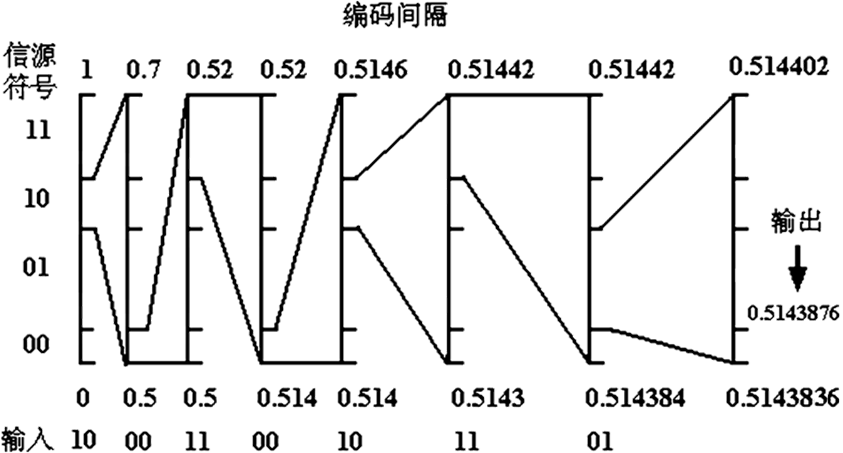## Arithmetic Coding Back

### Arithmetic Coding

• Arithmetic編碼: 是一種比Huffman編碼還要有效的現代編碼
• 浮點數精度需要考慮
• 用比例縮放來解決溢出問題
• 廣泛應用於圖像數據編碼#### 數據結構

``````weight;    //weight for "00", weight for "01"
//weight for "10", weight for "11"
``````

#### 算法實現

``````/* Code */
long double ARITHMETIC_CODING(char* input)
{
long double start = 0;
long double end = 1;
char extract;
if (strlen(input) % 2 != 0)
return -1.0;
else
{
char* ch = input;
while (*ch != '\0')
{
extract = *ch++;
extract = *ch++;
extract = '\0';
char* extract_string = extract;
if (!strcmp(extract_string, "11"))
{
start = end - (end - start) * weight;
}
else if (!strcmp(extract_string, "10"))
{
long double e = end - (end - start) * weight;
long double s = end - (end - start) * (weight + weight);
end = e;
start = s;
}
else if (!strcmp(extract_string, "01"))
{
long double e = end - (end - start) * (weight + weight);
long double s = start + (end - start) * (weight);
end = e;
start = s;
}
else
{    //"00"
end = start + (end - start) * (weight);
}
cout.precision(20);                                        //设置精度，防止损失
printf("start: %.12lf, end: %.12lf\n", start, end);
}
return (start + end) / 2;
}
}
``````